## Biomedical Research

All submissions of the EM system will be redirected to Online Manuscript Submission System. Authors are requested to submit articles directly to Online Manuscript Submission System of respective journal.

Research Article - Biomedical Research (2016) Volume 27, Issue 4

## RBC deformation analyzing within capillary blood flow using FSI method

Pedram Tehrani1*, Shahrokh Shojaei2

1Department of Mechanical Engineering, Islamic Azad University, Central Tehran Branch , Tehran, Iran

2Department of Biomedical Engineering, Islamic Azad University, Central Tehran Branch , Tehran, Iran

*Corresponding Author:
Pedram Tehrani
Department of Mechanical Engineering
Iran

Accepted date: March 07, 2016

Visit for more related articles at Biomedical Research

### Abstract

The purpose of this study is to investigate the behavior of red blood cells in order to pass through capillaries. In this research, the behavior of a single and multiple red blood cells are numerically investigated using a fluid-structure interaction (FSI) method. For calculations and comparisons of the deformation, displacements and stresses, four object points were selected and two different boundary conditions were applied to the problem. In the first, uniform velocity flow was applied at the inlet of the capillary and in the second model, the oscillating pressure was set. Through these investigations, the result reveals that the deformation in triple configuration of RBCs is more essential, sine the presence of the two additional cells above and beneath the original cell, will change the regime of flow and add extra amount to turbulency. By noticing this fact, in the uniform velocity flow pattern the results are expectable and convincing, because the displacement of triple array is greater than the single shape. But in the oscillating pressure pattern, there is no significant difference between the displacements of two corresponding points at two configurations. This output is in contrast with the results of uniform velocity flow and real situations. It shows that the assumption of uniform velocity flow at the beginning of the capillary is more logical than the oscillating pressure.

## Keywords

RBC, Deformation, Capillary, Blood, Uniform velocity, Oscillating pressure, FSI method.

## Introduction

Red Blood Ccells (RBCs), also named erythrocytes, are the most common type of cells in human blood which deliver oxygen (O2) to the body tissues by means of blood flow through the circulatory system. They carry oxygen in the lungs or gills and release it into tissues while squeezing through capillaries in the body. The primary function of carrying oxygen becomes possible by hemoglobin which is a chemically complex protein. When the blood circulates in the lungs, hemoglobin becomes almost completely saturated with oxygen, making the blood bright red. As red cells perfuse the capillary beds of tissues and organs, oxygen is released from the hemoglobin into the tissues. The RBC which is flowing in our body occupies 40-60% by volume ratio of blood (hematocrit), and exists in the capillaries with the large number. Human erythrocytes (red blood cells, RBCs) have a biconcave shape with an average diameter of ~8 mm [1-7]. During their 120-day life span, flowing through the human blood circulation system half a million times, RBCs experience large deformations in order to pass through capillaries as small as 3 μm in diameter [8,9]. As for the analysis of the flow phenomenon of the RBC the numerical simulation [10,11] as well as experiment observation  is becoming a strong tool. Particle methods, such as SPH method  and the MPS method  assumes both solid and liquid as particles, and can be used in complicated flow analysis. In case of using a particle method for the flow analysis of RBC, RBC is divided into the elastic film and internal liquid, and its deformation was investigated in detail . The capability of RBCs to experience transient deformations is one of the most important factors affecting their ability to circulate . Their high deformability is determinant for the passage or occlusion of capillary narrowing’s or stenosis, which are several times smaller than the RBCs [17,18]. Theoretical models have been widely used to investigate the shape transformations and the energy characteristics of free RBCs [19,20], RBCs under external forces [21,22]. They have also been used for studying the shape transformations and the energy characteristics of RBCs in a flow, principally for vessels which their radii are larger than the radii of the capillaries . These models predict that the RBCs are mainly distributed in the central region of the vessel, whereas there is a zone without cells in the vicinity of the vessel walls . Furthermore, in the microvasculature RBCs reach a symmetric, parachute-like shape or a nonsymmetric, slipper-like shape [24-26]. However, when the flow velocity is decreased, the parachute shape reverts to a discocyte shape [24,27]. Many researchers have investigated both simulation of fluid-structure interaction and experimental deformation of blood cells. Lim et al. has worked on the various experimental techniques for the investigation of biomechanics of single cell and molecule  and specifically the deformation of living cells via laser traps . The study also involved an actual three-dimensional finite element model of a human red blood cell and extensive computational investigation using ANSYS were carried out on the model. The finite element model is consistent with the experimental results under direct stretching of the cell in case of using laser tweezers. Eggleton and Popel  investigated Large deformation of red blood cells in shear flow by simulation via the immersed boundary method while elastic membranes with varying edge constraints and Young’s moduli has been investigated by means of ANSYS by Yang et al. . Jeong et al.  have measured the red blood cell deformation and velocity through capillaries with a diameter smaller than that of resting red blood cell in vivo by using high speed cameras to capture images of blood flow in capillaries and then estimating the deformation and velocity . An in depth explanation of the theory and application of the use of Arbitrary Lagrangian- Eulerian (ALE) Methods have also been studied by J. Donea et al. , which explains the advantages of the ALE method in fluid dynamics and nonlinear solid mechanics . Fluid-solid interaction (FSI) method is a powerful tool to study the deformation and stresses applied on the cell membrane. Therefore, in this study we developed a numerical approach by fluid-structure interaction to investigate the behaviour of a single and multiple red blood cells in a capillary.

## Method

Modeling of capillary and RBCs

A two- dimensional model was adopted for biconcave shape of RBCs with details of its geometry (Figure 1) which is located between the parallel rigid plates that form vessel walls, as shown in Figure 2. The model includes of elastic particles for the RBCs membrane, fluid particles for the blood plasma and rigid particles for the vessel walls. Two model configurations were used for our investigation. In the first model, a single RBC cell is placed at the center of the capillary as appeared in Figure 2. Pure shear stress which is resulted by the fluid flow is responsible for deformation of this single RBC. In the second model, a triple array of RBCs is considered as shown in Figure 3. In this circumstance, the interaction of the RBCs is taken into account and will be determinant in flow regime and consequently affects the magnitude of shear stress. In other words, deformation of RBCs is affected by cells interaction. According to the previous studies [35,36], the approximate diameter of the capillary is in the range of 4 to 20 mm. Therefore, the average diameter of 10 mm was used in this research. For calculations and comparisons of the deformation, displacements and stresses, four object points were selected. These points as shown in Figure 4 are the critical places that are determinant in our analysis.

Boundary conditions

Two different boundary conditions were applied to the problem. In the first, uniform velocity flow was applied at the inlet of the vessel. The Reynolds number in this situation was set to 0.001 . In the second model, the oscillating pressure was set at the inlet of the capillary. A sinusoidal pressure function with the amplitude of 20 mmHg and the frequency of 1 Hz was chosen according to Breyiannis’, G., and Pozrikidis’ study . In both cases, fluid-structure interaction (FSI) method was used for modeling the ties between the fluid and cell membrane. Furthermore, zero pressure and the non-slip condition were applied at the outlet and the inner vessel walls, respectively.

Equations

The first principle equation that has been used is the continuity. In general, the conservation of mass is expressed by the Equation 1.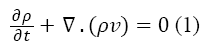Where the ρ is the density and v is the velocity of the fluid. Because the plasma is incompressible, Equation 1 is rewritten as below: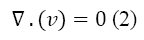The conservation of momentum in general is represented as Equation 3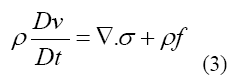Where represents the stress tensor, f is referred to body force such as gravity and the material derivative operator D/Dt is defined by Equation 4The kinematic condition or displacement compatibility is one of the fundamental conditions in the interface [38-40], which is given as:(5)

In above equation, df and ds represent the fluid and structure displacements, respectively.

Another necessary condition for the interface is dynamic condition  as below: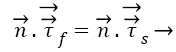(6)

Where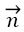is unit normal vector and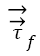and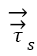represents stresses of fluid and solid, respectively. These equations 1-6 are numerically solved and used for calculation of the deformations, displacements and stresses of the RBCs.

Simulation was performed using fully coupled fluid structure interaction solving capability of commercial software ADINA 8.8. For the solid and fluid model, the number of iterations and iteration tolerance is set to 1600 (each step equal to 0.001) and 0.001, respectively. All computations in this research were performed on a mesh system of 9726 cells for the fluid domain and1258 cells for the solid domain in single RBC modeling and 7610 cells for the fluid domain and 4017 cells for the solid domain in triple RBCs configuration. It is worth mentioning that the central processing unit (CPU) time of the system is about 3 times longer than that of the adopted mesh system.

## Results and Discussion

The results for uniform velocity flow for single RBC and triple RBCs are shown in Figures 5-9. Figure 5 represents the stress on four selected points in single configuration of RBC. As it is shown, the point 2 has the maximum magnitude of stress and point 1 and 3 have the same corresponding stresses. Figure 6, shows the deformations of these points in single array. Deformation of single RBC and triple RBCs in uniform velocity flow are shown in Figure 7 and Figure 8. In Figure 9 the stresses at two corresponding points (point 2), in single and triple arrays are compared. As it is shown, the stress in triple configuration is higher than the single one.

Figure 9: Comparison of stresses in single and triple arrays. 1 is related to single and 3 to triple.

Due to the shear stress in triple arrays is much more than the single one, the percentage of hemolysis is increase. The results for Oscillating pressure flow for single RBC and triple RBCs are shown in Figures 10 and 11. As it is stated, a sinusoidal pressure was applied to the entrance of the vessel. Figure 10 shows the displacement of the single RBC. Just like the uniform velocity flow, point 2 has the greatest displacement and the curve of points 1 and 3 coincide. A comparison of the displacements of analogous points in single and triple configurations is shown in Figure 11. It is obvious that in contrast with uniform velocity flow, the displacements in two cases have comparable magnitudes.

Figure 11: Comparison of displacement (m) in single and triple arrays. 1 is related to single and 3 to triple

## Conclusions

In all the cases, as shown in previous figures, point 2 has the maximum amounts of displacement, deformation and stress as previous works [17,18]. Point 2 is the first location that the particles of plasma collide with the RBC cell and it acts as stagnation point. It is logical that maximum stress appear at this point. Point 1 and 3 are symmetric in a non-deformed RBC. This symmetric shape applies that these 2 points should have same displacements and inverse deformations, as it is achieved by calculations. It is reasonable that we expect more essential deformation in triple configuration of RBCs, sine the presence of the two additional cells above and beneath the original cell will change the regime of flow and add extra amount to turbulency. By noticing this fact, in the uniform velocity flow pattern the results are expectable and convincing, because the displacement of triple array is greater than the single shape. But in the oscillating pressure pattern, there is no significant difference between the displacements of two corresponding points at two configurations. This output is in contrast with the results of uniform velocity flow and real situations. It shows that the assumption of uniform velocity flow at the beginning of the capillary is more logical than the oscillating pressure.